# 18. Linked lists¶

## 18.1. Embedded references¶

We have seen examples of attributes that refer to other objects, which we called embedded references. A common data structure, the linked list, takes advantage of this feature.

Linked lists are made up of nodes, where each node contains a reference to the next node in the list. In addition, each node contains a unit of data called the cargo.

A linked list is considered a recursive data structure because it has a recursive definition.

A linked list is either:

1. the empty list, represented by `None`, or
2. a node that contains a cargo object and a reference to a linked list.

Recursive data structures lend themselves to recursive methods.

## 18.2. The `Node` class¶

As usual when writing a new class, we’ll start with the initialization and `__str__` methods so that we can test the basic mechanism of creating and displaying the new type:

```class Node(object):
def __init__(self, cargo=None, next=None):
self.cargo = cargo
self.next  = next

def __str__(self):
return str(self.cargo)
```

As usual, the parameters for the initialization method are optional. By default, both the cargo and the link, `next`, are set to `None`.

The string representation of a node is just the string representation of the cargo. Since any value can be passed to the `str` function, we can store any value in a list.

We could also write getters and setters for the `cargo` and `next` instance variables:

```class Node(object):
...
...
def getCargo(self):
return self.cargo
def getNext(self):
return self.next

def setCargo(self,c):
self.cargo = c
def setNext(self,n):
self.next = n
```

To test the implementation so far, we can create a `Node` and print it:

```>>> node = Node("test")
>>> print(node)
test
>>> print(node.getNext())
None
```

To make it interesting, we need create more than one node, and link them together:

```>>> node1 = Node(1)
>>> node2 = Node(2)
>>> node3 = Node(3)
```

This code creates three nodes, but we don’t have a linked-list yet because the nodes are not linked. The state diagram looks like this: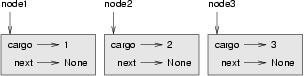To link the nodes, we have to make the first node refer to the second and the second node refer to the third:

```>>> node1.setNext(node2)
>>> node2.setNext(node3)
```

The reference of the third node is `None`, which indicates that it is the end of the list. Now the state diagram looks like this: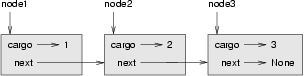Now you know how to create nodes and link them together. What might be less clear at this point is why.

## 18.3. Lists as collections¶

Lists are useful because they provide a way to assemble multiple objects into a single entity, sometimes called a collection. In the example, the first node of the list serves as a reference to the entire list.

To pass the list as a parameter, we only have to pass a reference to the first node. For example, the function `print_list` takes a single node as an argument (`curr`, short for current). Assuming that is the head of the list, is accumulates the cargo from each node, printing out the contents of the list at the end:

```def print_list(curr):
output = ""
while curr:
output += str(curr) + " "
curr = curr.getNext()
print(output)
```

To invoke this method, we pass a reference to the first node:

```>>> print_list(node1)
1 2 3
```

Inside `print_list` we have a reference to the first node of the list, but there is no variable that refers to the other nodes. We have to use the `next` value from each node to get to the next node.

To traverse a linked list, it is common to use a loop variable like `curr` to refer to each of the nodes in succession.

This diagram shows the full list and the values that `curr` takes on: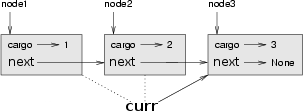## 18.4. Lists and recursion¶

It is natural to express many list operations using recursive methods. For example, the following is a recursive algorithm for printing a list backwards:

1. Separate the list into two pieces: the first node (called the head); and the rest (called the tail).
2. Print the tail backward.
3. Print the head.

Of course, Step 2, the recursive call, assumes that we have a way of printing a list backward. But if we assume that the recursive call works – the leap of faith – then we can convince ourselves that this algorithm works.

All we need are a base case and a way of proving that for any list, we will eventually get to the base case. Given the recursive definition of a list, a natural base case is the empty list, represented by `None`.

```def print_backward_helper(curr):
if curr == None:
return ""
else:
tail = curr.getNext()
return print_backward_helper(tail) + str(head) + " "

def print_backward(firstnode):
output = print_backward_helper(firstnode)
print(output)
```

In the above example, we use a helper function, so we can just call `print_backward(node1)` the same as we called `print_list(node1)`. The helper function does the actual recursion and accumulating of the string. The first line of the helper function handles the base case by returning an empty string (and not recurring). The else case (if we are not at the end of the list) splits the list into `head` and `tail`. The final return line adds the head cargo and a space to the reversed rest of the list.

We invoke this method as we invoked `print_list`:

```>>> print_backward(node1)
3 2 1
```

The result is the list contents printed in reverse.

You might wonder why `print_list` and `print_backward` are functions and not methods in the `Node` class. The reason is that we want to use `None` to represent the empty list and it is not legal to invoke a method on `None`. This limitation makes it awkward to write list-manipulating code in a clean object-oriented style.

Can we prove that `print_backward` will always terminate? In other words, will it always reach the base case? In fact, the answer is no. Some lists will make this method crash.

## 18.5. Infinite lists¶

There is nothing to prevent a node from referring back to an earlier node in the list, including itself. For example, this figure shows a list with two nodes, one of which refers to itself: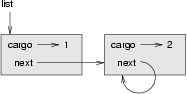If we invoke `print_list` on this list, it will loop forever. If we invoke `print_backward`, it will recurse infinitely. This sort of behavior makes infinite lists difficult to work with.

Nevertheless, they are occasionally useful. For example, we might represent a number as a list of digits and use an infinite list to represent a repeating fraction.

Regardless, it is problematic that we cannot prove that `print_list` and `print_backward` terminate. The best we can do is the hypothetical statement, If the list contains no loops, then these methods will terminate. This sort of claim is called a precondition. It imposes a constraint on one of the parameters and describes the behavior of the method if the constraint is satisfied. You will see more examples soon.

## 18.6. The fundamental ambiguity theorem¶

One part of `print_backward` might have raised an eyebrow:

```head = curr
tail = curr.getNext()
```

After the first assignment, `head` and `curr` have the same type and the same value. So why did we create a new variable?

The reason is that the two variables play different roles. We think of `head` as a reference to a single node, and we think of `curr` as a reference to the first node of a list. These roles are not part of the program; they are in the mind of the programmer.

In general we can’t tell by looking at a program what role a variable plays. This ambiguity can be useful, but it can also make programs difficult to read. We often use variable names like `node` and `curr` to document how we intend to use a variable and sometimes create additional variables to disambiguate.

We could have written `print_backward_helper` without `head` and `tail`, which makes it more concise but possibly less clear:

```def print_backward_helper(curr):
if curr == None: return ""
return print_backward_helper(curr.getNext()) + str(curr) + " "
```

The fundamental ambiguity theorem describes the ambiguity that is inherent in a reference to a node: A variable that refers to a node might treat the node as a single object or as the first in a list of nodes.

## 18.7. Modifying lists¶

There are two ways to modify a linked list. Obviously, we can change the cargo of one of the nodes, but the more interesting operations are the ones that add, remove, or reorder the nodes.

As an example, let’s write a method that removes the second node in the list and returns a reference to the removed node:

```def removeSecond(lst):
if lst == None: return
first = lst
second = lst.getNext()
# make the first node refer to the third
first.setNext(second.getNext())
# separate the second node from the rest of the list
second.setNext(None)
return second
```

Again, we are using temporary variables to make the code more readable. Here is how to use this method:

```>>> print_list(node1)
1 2 3
>>> removed = removeSecond(node1)
>>> print_list(removed)
2
>>> print_list(node1)
1 3
```

This state diagram shows the effect of the operation: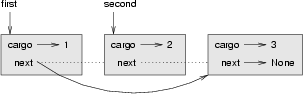What happens if you invoke this method and pass a list with only one element (a singleton)? What happens if you pass the empty list as an argument? Is there a precondition for this method? If so, fix the method to handle a violation of the precondition in a reasonable way.

## 18.8. Wrappers and helpers¶

It is often useful to divide a list operation into two methods, as we did above with the helper function. As an addition to that example, printing a list backward in the conventional list format `[3, 2, 1]` we can simply add code to the `print_backward` method:

```def print_backward(firstnode):
output = print_backward_helper(firstnode)
lstbkwd = "[" + output[:len(output)-2] + "]"
print(lstbkwd)
```

Again, it is a good idea to check methods like this to see if they work with special cases like an empty list or a singleton.

When we use this method, `print_backward` acts as a wrapper, and it uses `print_backward_helper` as a helper.

## 18.9. The `LinkedList` class¶

There are some subtle problems with the way we have been implementing lists. In a reversal of cause and effect, we’ll propose an alternative implementation first and then explain what problems it solves.

First, we’ll create a new class called `LinkedList`. Its attributes are an integer that contains the length of the list and a reference to the first node. `LinkedList` objects serve as handles for manipulating lists of `Node` objects:

```class LinkedList(object):
def __init__(self):
self.length = 0
```

One nice thing about the `LinkedList` class is that it provides a natural place to put wrapper functions like `print_backward`, which we can make a method of the `LinkedList` class:

```class LinkedList(object):
...
def print_backward_helper(self,curr):
if curr == None:
return ""
else:
tail = curr.getNext()
return self.print_backward_helper(tail) + str(head) + ", "

def print_backward(self):
lstbkwd = "[" + output[:len(output)-2] + "]"
print(lstbkwd)
```

Another benefit of the `LinkedList` class is that it makes it easier to add or remove the first element of a list. For example, `addFirst` is a method for `LinkedList`s; it takes an item of cargo as an argument and puts it at the beginning of the list:

```class LinkedList(object):
...
node = Node(cargo)
self.length = self.length + 1
```

As usual, you should check code like this to see if it handles the special cases. For example, what happens if the list is initially empty?

## 18.10. Invariants¶

Some lists are well formed ; others are not. For example, if a list contains a loop, it will cause many of our methods to crash, so we might want to require that lists contain no loops. Another requirement is that the `length` value in the `LinkedList` object should be equal to the actual number of nodes in the list.

Requirements like these are called invariants because, ideally, they should be true of every object all the time. Specifying invariants for objects is a useful programming practice because it makes it easier to prove the correctness of code, check the integrity of data structures, and detect errors.

One thing that is sometimes confusing about invariants is that there are times when they are violated. For example, in the middle of `addFirst`, after we have added the node but before we have incremented `length`, the invariant is violated. This kind of violation is acceptable; in fact, it is often impossible to modify an object without violating an invariant for at least a little while. Normally, we require that every method that violates an invariant must restore the invariant.

If there is any significant stretch of code in which the invariant is violated, it is important for the comments to make that clear, so that no operations are performed that depend on the invariant.

## 18.11. Glossary¶

embedded reference
A reference stored in an attribute of an object.
A data structure that implements a collection using a sequence of linked nodes.
node
An element of a list, usually implemented as an object that contains a reference to another object of the same type.
cargo
An item of data contained in a node.
An embedded reference used to link one object to another.
precondition
An assertion that must be true in order for a method to work correctly.
fundamental ambiguity theorem
A reference to a list node can be treated as a single object or as the first in a list of nodes.
singleton
A linked list with a single node.
wrapper
A method that acts as a middleman between a caller and a helper method, often making the method easier or less error-prone to invoke.
helper
A method that is not invoked directly by a caller but is used by another method to perform part of an operation.
invariant
An assertion that should be true of an object at all times (except perhaps while the object is being modified).

## 18.12. Exercises¶

1. By convention, lists are often printed in brackets with commas between the elements, as in `[1, 2, 3]`. Modify `print_list` so that it generates output in this format.